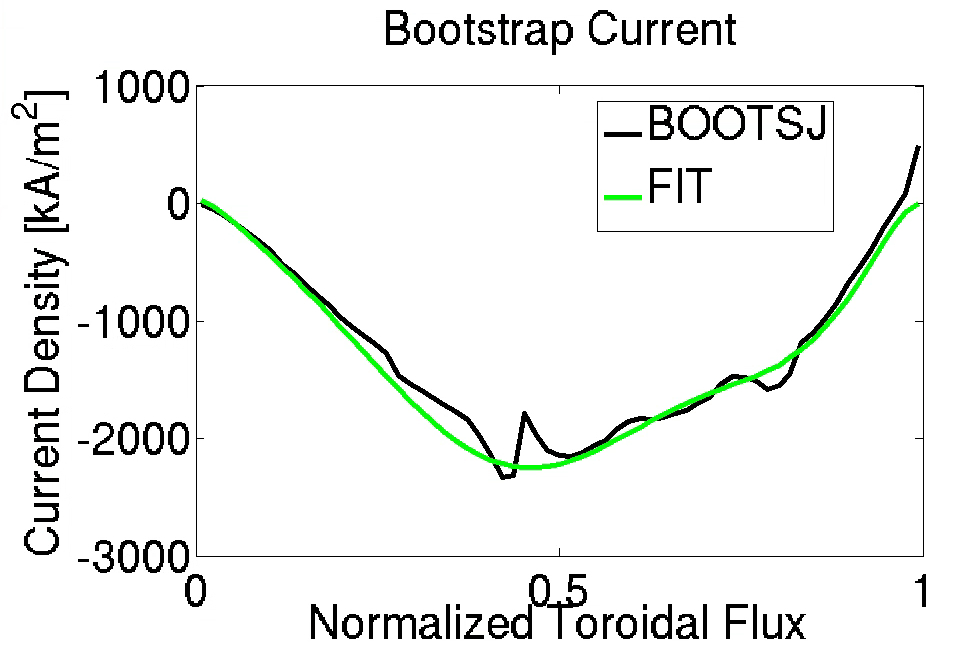# STELLOPT

State-of-the-art stellarator optimization code

# BOOTSJ

<<toc>>This code calculates the bootstrap current for 3D magnetic equilibria provided by VMEC.

### Theory

The code BOOTSJ<ref>K.C. Shaing, B.A. Carreras, N. Dominguez, V.E. Lynch, J.S. Tolliver 1989 Phys. Fluids B 1 1663</ref><ref>K.C. Shaing, E.C. Crume, J.S. Tolliver, S.P. Hirshman, W.I. van Rij 1989 Phys. Fluids B 1 148</ref><ref>K.C. Shaing, S.P. Hirshman, J.S. Tolliver 1986 *Phys. Fluids * 29 2548</ref> calculates the bootstrap current for 3D VMEC equilibria.

### Compilation

VMEC is a component of the STELLOPT suite of codes. It is contained within the 'stellopt.zip' file. Compilation of the STELLOPT suite is discussed on the STELLOPT Compilation Page.

### Input Data Format

The BOOTSJ code takes in input file from the command line. The first line of the input file is the VMEC extension for a given VMEC equilibria. The next line (or multiple lines) are a space delimited list of surfaces on which to calculate the bootstrap current. The BOOTSJ code requires both an VMEC equilibrium and the output the from BOOZER_XFORM code (transformation to boozer coordinates). Additionally, the VMEC input file must contain an additional namelist BOOTIN. The BOOTIN namelist reads as follows

#!fortran
&BOOTIN
!  NRHO is obsolete
NRHO=0
!  Number of poloidal modes
MBUSE=0
!  Number of toroidal modes
NBUSE=0
!  Effective Ion Charge
ZEFF1=1.000000000000000E+00
!  Damping factor for resonance
DAMP_BS=-1.000000000000000
! Temperature/Density Data
!    ATE   : Electron temperature (10th order polynomial, keV)
!    ATI   : Ion temperature (10th order polynomial, keV)
!    TEMPRES:  Scale temperatures as P^tempres (if tempres >=0)
!    TETI  : Temperature ratio Te/Ti (if tempres >=0)
!    DENS0 : Central electron density in 10^20 [m^-3] (if tempres >=0)
! Electron Temperature (10th order polynomial, keV)
ATE=0.000000000000000E+00  0.000000000000000E+00  0.000000000000000E+00
0.000000000000000E+00  0.000000000000000E+00  0.000000000000000E+00
0.000000000000000E+00  0.000000000000000E+00  0.000000000000000E+00
0.000000000000000E+00  0.000000000000000E+00
ATI=0.000000000000000E+00  0.000000000000000E+00  0.000000000000000E+00
0.000000000000000E+00  0.000000000000000E+00  0.000000000000000E+00
0.000000000000000E+00  0.000000000000000E+00  0.000000000000000E+00
0.000000000000000E+00  0.000000000000000E+00
TEMPRES = -1.0  ! Set to 1 to use P profile for te/ti
TETI    = 1.0
DENS0   = 1.0
/


If the leading coefficient of either ATE or ATI is negative, the code assumes maximum density and then calculate the profiles using a power law given by abs(TEMPRES). The TEMPRES coefficient must be negative and cannot be greater than one. In this instance the profiles are determined by DENS0 and TEMPRES. If the user does not specify TEMPRES in the input namelist it defaults to -1 and the code assume ATE and ATI are the 10th order polynomials for the electron and ion temperature respectively (TETI is ignored).

For example if the user wished to calculate the bootstrap current on the surfaces 2, 5, and 37 for a VMEC run with extension 'test' the input file would look like:

#!fortran
test
2 5 37


### Execution

The BOOTSJ code is executed from the command line by specifying the name of the input file (described above). The user may suppress screen output by passing the F flag at the command line. To execute the code with a input file names in_bootsj.test the command would look like:

> xbootsj in_bootsj.test


### Output Data Format

The BOOTSJ code outputs three text files jBds.ext, answers.ext, and answers_plot.ext where 'ext' is the same extension as the VMEC equilibria. The jBds and answers file contain text headers explaining the meaning of each column of data. The answers_plot file contains columns of data where each row is a different surface. The columns in order are:

R  GNORM  AMAIN  LAMINT  OTHER  dI/ds  J_GRAD_NE  J_GRAD_NI  J_GRAD_TE  J_GRAD_TI  Q  FTRAPPED  <Jbs>/<Jbs-tok>  TE  TI  NE  NE/Zeff  BETA  <Jbs_dot_B>


The column dI/ds is the current density profile in units normalized to mu0. The total current is the sum of this column divided by mu0=4*pi*1E-6.

### Visualization

Output are text files which can be read in with many plotting packages.

### Tutorials

Bootstrap Calculation for NCSX-Like configuration